# Source reconstruction using an LCMV beamformer#

This tutorial gives an overview of the beamformer method and shows how to reconstruct source activity using an LCMV beamformer.

```# Authors: Britta Westner <britta.wstnr@gmail.com>
#          Eric Larson <larson.eric.d@gmail.com>
#
```
```import matplotlib.pyplot as plt
import mne
from mne.datasets import sample, fetch_fsaverage
from mne.beamformer import make_lcmv, apply_lcmv
```

## Introduction to beamformers#

A beamformer is a spatial filter that reconstructs source activity by scanning through a grid of pre-defined source points and estimating activity at each of those source points independently. A set of weights is constructed for each defined source location which defines the contribution of each sensor to this source. Beamformers are often used for their focal reconstructions and their ability to reconstruct deeper sources. They can also suppress external noise sources. The beamforming method applied in this tutorial is the linearly constrained minimum variance (LCMV) beamformer 1 operates on time series. Frequency-resolved data can be reconstructed with the dynamic imaging of coherent sources (DICS) beamforming method 2. As we will see in the following, the spatial filter is computed from two ingredients: the forward model solution and the covariance matrix of the data.

## Data processing#

We will use the sample data set for this tutorial and reconstruct source activity on the trials with left auditory stimulation.

```data_path = sample.data_path()
subjects_dir = data_path / "subjects"
meg_path = data_path / "MEG" / "sample"
raw_fname = meg_path / "sample_audvis_filt-0-40_raw.fif"

# Set up the epoching
event_id = 1  # those are the trials with left-ear auditory stimuli
tmin, tmax = -0.2, 0.5
events = mne.find_events(raw)

# pick relevant channels
raw.pick(["meg", "eog"])  # pick channels of interest

# Create epochs
proj = False  # already applied
epochs = mne.Epochs(
raw,
events,
event_id,
tmin,
tmax,
baseline=(None, 0),
proj=proj,
)

# for speed purposes, cut to a window of interest
evoked = epochs.average().crop(0.05, 0.15)

# Visualize averaged sensor space data
evoked.plot_joint()

del raw  # save memory
```
•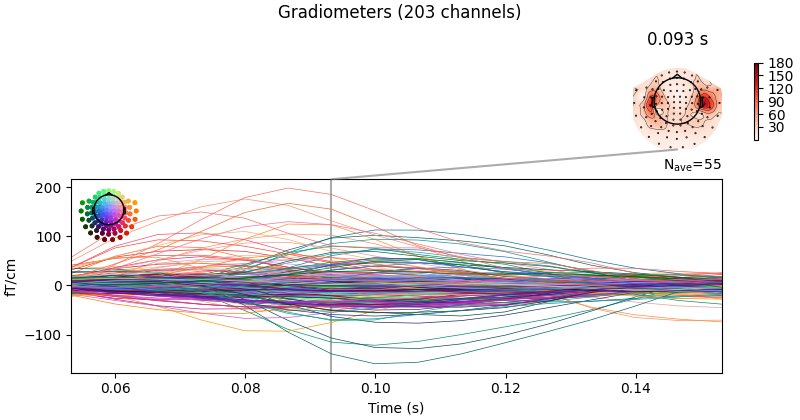•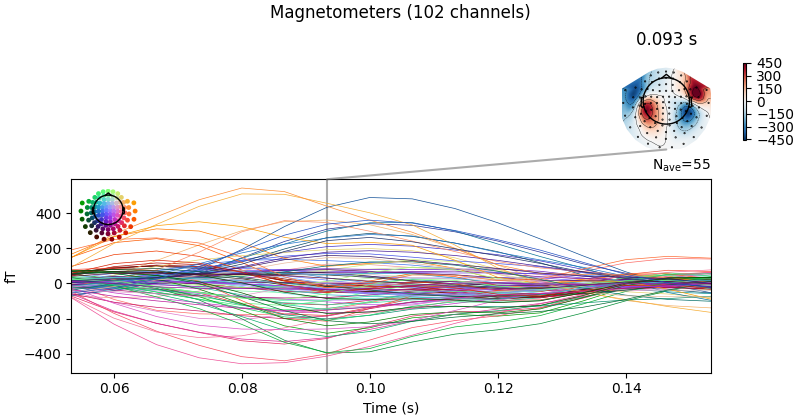```Opening raw data file /home/circleci/mne_data/MNE-sample-data/MEG/sample/sample_audvis_filt-0-40_raw.fif...
Read a total of 4 projection items:
PCA-v1 (1 x 102)  idle
PCA-v2 (1 x 102)  idle
PCA-v3 (1 x 102)  idle
Average EEG reference (1 x 60)  idle
Range : 6450 ... 48149 =     42.956 ...   320.665 secs
319 events found
Event IDs: [ 1  2  3  4  5 32]
Removing projector <Projection | Average EEG reference, active : False, n_channels : 60>
72 matching events found
Setting baseline interval to [-0.19979521315838786, 0.0] s
Applying baseline correction (mode: mean)
Created an SSP operator (subspace dimension = 3)
Rejecting  epoch based on EOG : ['EOG 061']
Rejecting  epoch based on EOG : ['EOG 061']
Rejecting  epoch based on EOG : ['EOG 061']
Rejecting  epoch based on EOG : ['EOG 061']
Rejecting  epoch based on EOG : ['EOG 061']
Rejecting  epoch based on MAG : ['MEG 1711']
Rejecting  epoch based on EOG : ['EOG 061']
Rejecting  epoch based on EOG : ['EOG 061']
Rejecting  epoch based on EOG : ['EOG 061']
Rejecting  epoch based on EOG : ['EOG 061']
Rejecting  epoch based on EOG : ['EOG 061']
Rejecting  epoch based on EOG : ['EOG 061']
Rejecting  epoch based on EOG : ['EOG 061']
Rejecting  epoch based on EOG : ['EOG 061']
Rejecting  epoch based on EOG : ['EOG 061']
Rejecting  epoch based on EOG : ['EOG 061']
Rejecting  epoch based on EOG : ['EOG 061']
NOTE: pick_channels() is a legacy function. New code should use inst.pick(...).
Created an SSP operator (subspace dimension = 3)
3 projection items activated
SSP projectors applied...
NOTE: pick_channels() is a legacy function. New code should use inst.pick(...).
NOTE: pick_channels() is a legacy function. New code should use inst.pick(...).
Removing projector <Projection | PCA-v1, active : True, n_channels : 102>
Removing projector <Projection | PCA-v2, active : True, n_channels : 102>
Removing projector <Projection | PCA-v3, active : True, n_channels : 102>
NOTE: pick_channels() is a legacy function. New code should use inst.pick(...).
NOTE: pick_channels() is a legacy function. New code should use inst.pick(...).
NOTE: pick_channels() is a legacy function. New code should use inst.pick(...).
```

## Computing the covariance matrices#

Spatial filters use the data covariance to estimate the filter weights. The data covariance matrix will be inverted during the spatial filter computation, so it is valuable to plot the covariance matrix and its eigenvalues to gauge whether matrix inversion will be possible. Also, because we want to combine different channel types (magnetometers and gradiometers), we need to account for the different amplitude scales of these channel types. To do this we will supply a noise covariance matrix to the beamformer, which will be used for whitening. The data covariance matrix should be estimated from a time window that includes the brain signal of interest, and incorporate enough samples for a stable estimate. A rule of thumb is to use more samples than there are channels in the data set; see 34 for more detailed advice on covariance estimation for beamformers. Here, we use a time window incorporating the expected auditory response at around 100 ms post stimulus and extend the period to account for a low number of trials (72) and low sampling rate of 150 Hz.

```data_cov = mne.compute_covariance(epochs, tmin=0.01, tmax=0.25, method="empirical")
noise_cov = mne.compute_covariance(epochs, tmin=tmin, tmax=0, method="empirical")
data_cov.plot(epochs.info)
del epochs
```
•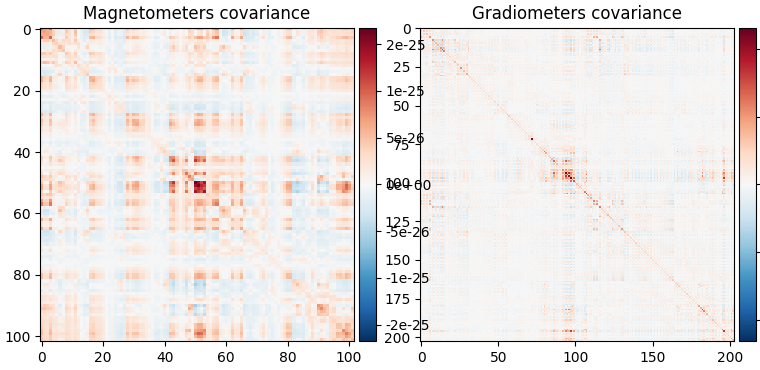•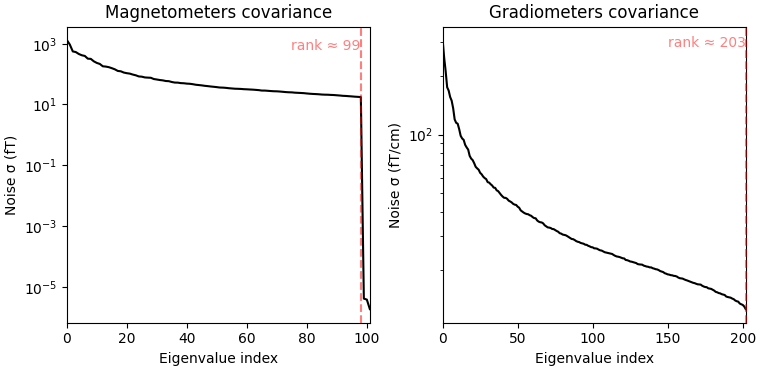```Computing rank from data with rank=None
Using tolerance 4.1e-09 (2.2e-16 eps * 305 dim * 6.1e+04  max singular value)
Estimated rank (mag + grad): 302
MEG: rank 302 computed from 305 data channels with 3 projectors
Created an SSP operator (subspace dimension = 3)
Setting small MEG eigenvalues to zero (without PCA)
Reducing data rank from 305 -> 302
Estimating covariance using EMPIRICAL
Done.
Number of samples used : 2035
[done]
Computing rank from data with rank=None
Using tolerance 2.8e-09 (2.2e-16 eps * 305 dim * 4.2e+04  max singular value)
Estimated rank (mag + grad): 302
MEG: rank 302 computed from 305 data channels with 3 projectors
Created an SSP operator (subspace dimension = 3)
Setting small MEG eigenvalues to zero (without PCA)
Reducing data rank from 305 -> 302
Estimating covariance using EMPIRICAL
Done.
Number of samples used : 1705
[done]
Computing rank from covariance with rank=None
Using tolerance 4e-14 (2.2e-16 eps * 102 dim * 1.8  max singular value)
Estimated rank (mag): 99
MAG: rank 99 computed from 102 data channels with 0 projectors
Computing rank from covariance with rank=None
Using tolerance 4.3e-13 (2.2e-16 eps * 203 dim * 9.4  max singular value)
GRAD: rank 203 computed from 203 data channels with 0 projectors
```

When looking at the covariance matrix plots, we can see that our data is slightly rank-deficient as the rank is not equal to the number of channels. Thus, we choose to regularize the covariance matrix before inverting it in the beamformer calculation. This can be achieved by setting the parameter `reg=0.05` when calculating the spatial filter with `make_lcmv()`. This corresponds to loading the diagonal of the covariance matrix with 5% of the sensor power. Other ways to deal with rank-deficient covariance matrices are discussed in 4.

## The forward model#

The forward model is the other important ingredient for the computation of a spatial filter. Here, we will load the forward model from disk; more information on how to create a forward model can be found in this tutorial: Head model and forward computation. Note that beamformers are usually computed in a `volume source space`, because estimating only cortical surface activation can misrepresent the data.

```# Read forward model

fwd_fname = meg_path / "sample_audvis-meg-vol-7-fwd.fif"
```
```Reading forward solution from /home/circleci/mne_data/MNE-sample-data/MEG/sample/sample_audvis-meg-vol-7-fwd.fif...
[done]
Desired named matrix (kind = 3523) not available
Read MEG forward solution (3757 sources, 306 channels, free orientations)
Source spaces transformed to the forward solution coordinate frame
```

## Handling depth bias#

The forward model solution is inherently biased toward superficial sources. When analyzing single conditions it is best to mitigate the depth bias somehow. There are several ways to do this:

• `mne.beamformer.make_lcmv()` has a `depth` parameter that normalizes the forward model prior to computing the spatial filters. See the docstring for details.

• Unit-noise gain beamformers handle depth bias by normalizing the weights of the spatial filter. Choose this by setting `weight_norm='unit-noise-gain'`.

• When computing the Neural activity index, the depth bias is handled by normalizing both the weights and the estimated noise (see 1). Choose this by setting `weight_norm='nai'`.

Note that when comparing conditions, the depth bias will cancel out and it is possible to set both parameters to `None`.

## Compute the spatial filter#

Now we can compute the spatial filter. We’ll use a unit-noise gain beamformer to deal with depth bias, and will also optimize the orientation of the sources such that output power is maximized. This is achieved by setting `pick_ori='max-power'`. This gives us one source estimate per source (i.e., voxel), which is known as a scalar beamformer.

```filters = make_lcmv(
evoked.info,
forward,
data_cov,
reg=0.05,
noise_cov=noise_cov,
pick_ori="max-power",
weight_norm="unit-noise-gain",
rank=None,
)

# You can save the filter for later use with:
# filters.save('filters-lcmv.h5')
```
```Computing rank from covariance with rank=None
Using tolerance 7.1e-13 (2.2e-16 eps * 305 dim * 10  max singular value)
Estimated rank (mag + grad): 302
MEG: rank 302 computed from 305 data channels with 3 projectors
Computing rank from covariance with rank=None
Using tolerance 2.9e-13 (2.2e-16 eps * 305 dim * 4.3  max singular value)
Estimated rank (mag + grad): 302
MEG: rank 302 computed from 305 data channels with 3 projectors
Making LCMV beamformer with rank {'meg': 302}
Computing inverse operator with 305 channels.
305 out of 306 channels remain after picking
Selected 305 channels
Whitening the forward solution.
Created an SSP operator (subspace dimension = 3)
Computing rank from covariance with rank={'meg': 302}
Setting small MEG eigenvalues to zero (without PCA)
Creating the source covariance matrix
Computing beamformer filters for 3757 sources
Filter computation complete
```

It is also possible to compute a vector beamformer, which gives back three estimates per voxel, corresponding to the three direction components of the source. This can be achieved by setting `pick_ori='vector'` and will yield a `volume vector source estimate`. Note that we switch the `weight_norm` parameter to `'unit-noise-gain-invariant'`, which is only necessary for the vector unit-noise-gain beamformer. For more in-depth detail, see 4. We will compute another set of filters using the vector beamformer approach:

```filters_vec = make_lcmv(
evoked.info,
forward,
data_cov,
reg=0.05,
noise_cov=noise_cov,
pick_ori="vector",
weight_norm="unit-noise-gain-invariant",
rank=None,
)
# save a bit of memory
src = forward["src"]
del forward
```
```Computing rank from covariance with rank=None
Using tolerance 7.1e-13 (2.2e-16 eps * 305 dim * 10  max singular value)
Estimated rank (mag + grad): 302
MEG: rank 302 computed from 305 data channels with 3 projectors
Computing rank from covariance with rank=None
Using tolerance 2.9e-13 (2.2e-16 eps * 305 dim * 4.3  max singular value)
Estimated rank (mag + grad): 302
MEG: rank 302 computed from 305 data channels with 3 projectors
Making LCMV beamformer with rank {'meg': 302}
Computing inverse operator with 305 channels.
305 out of 306 channels remain after picking
Selected 305 channels
Whitening the forward solution.
Created an SSP operator (subspace dimension = 3)
Computing rank from covariance with rank={'meg': 302}
Setting small MEG eigenvalues to zero (without PCA)
Creating the source covariance matrix
Computing beamformer filters for 3757 sources
Filter computation complete
```

## Apply the spatial filter#

The spatial filter can be applied to different data types: raw, epochs, evoked data or the data covariance matrix to gain a static image of power. The function to apply the spatial filter to `Evoked` data is `apply_lcmv()` which is what we will use here. The other functions are `apply_lcmv_raw()`, `apply_lcmv_epochs()`, and `apply_lcmv_cov()`.

```stc = apply_lcmv(evoked, filters)
stc_vec = apply_lcmv(evoked, filters_vec)
del filters, filters_vec
```

## Visualize the reconstructed source activity#

We can visualize the source estimate in different ways, e.g. as a volume rendering, an overlay onto the MRI, or as an overlay onto a glass brain.

The plots for the scalar beamformer show brain activity in the right temporal lobe around 100 ms post stimulus. This is expected given the left-ear auditory stimulation of the experiment.

```lims = [0.3, 0.45, 0.6]
kwargs = dict(
src=src,
subject="sample",
subjects_dir=subjects_dir,
initial_time=0.087,
verbose=True,
)
```

### On MRI slices (orthoview; 2D)#

```stc.plot(mode="stat_map", clim=dict(kind="value", pos_lims=lims), **kwargs)
```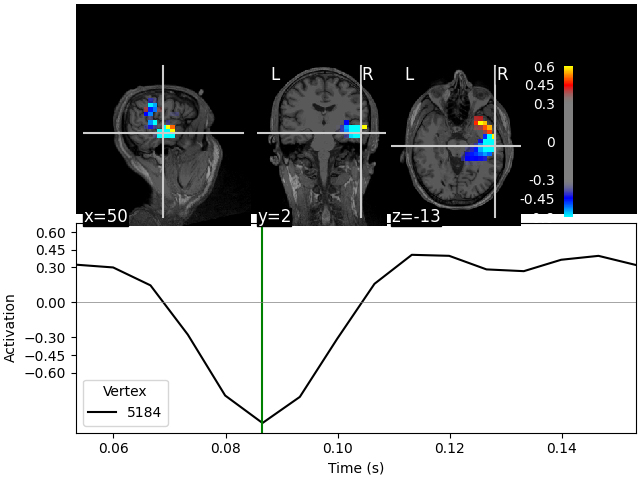```Fixing initial time: 0.087 s
Showing: t = 0.087 s, (50.7, 2.0, -13.3) mm, [18, 12, 9] vox, 5184 vertex
```

### On MNI glass brain (orthoview; 2D)#

```stc.plot(mode="glass_brain", clim=dict(kind="value", lims=lims), **kwargs)
```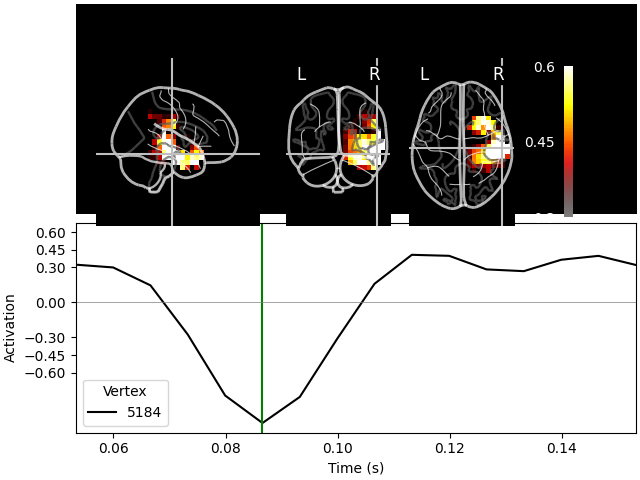```Transforming subject RAS (non-zero origin) -> MNI Talairach
1.022485 -0.008449 -0.036217       5.60 mm
0.071071  0.914866  0.406098     -19.82 mm
0.008756 -0.433700  1.028119      -1.55 mm
0.000000  0.000000  0.000000       1.00

Fixing initial time: 0.087 s
Showing: t = 0.087 s, (57.9, -19.7, -15.6) mm, [18, 12, 9] vox, 5184 vertex
```

### Volumetric rendering (3D) with vectors#

These plots can also be shown using a volumetric rendering via `plot_3d()`. Let’s try visualizing the vector beamformer case. Here we get three source time courses out per voxel (one for each component of the dipole moment: x, y, and z), which appear as small vectors in the visualization (in the 2D plotters, only the magnitude can be shown):

```brain = stc_vec.plot_3d(
clim=dict(kind="value", lims=lims),
hemi="both",
size=(600, 600),
views=["sagittal"],
# Could do this for a 3-panel figure:
# view_layout='horizontal', views=['coronal', 'sagittal', 'axial'],
brain_kwargs=dict(silhouette=True),
**kwargs
)
```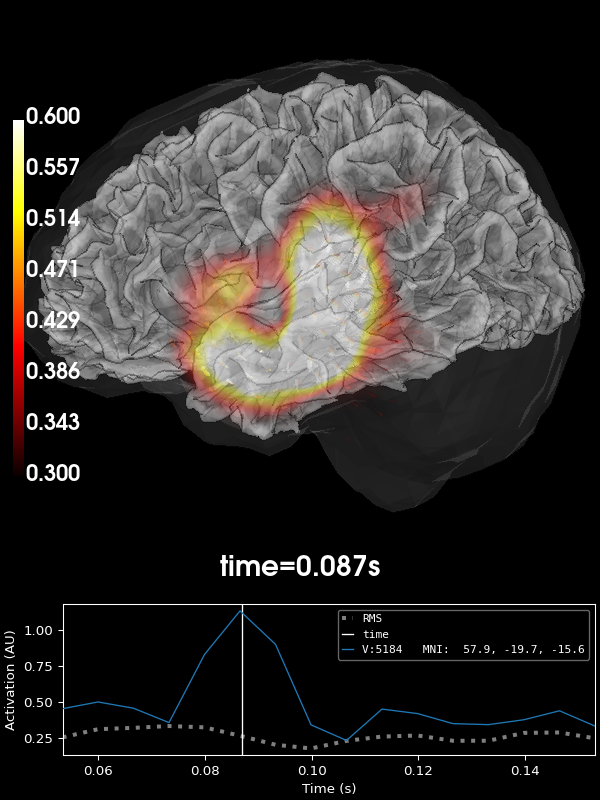## Visualize the activity of the maximum voxel with all three components#

We can also visualize all three components in the peak voxel. For this, we will first find the peak voxel and then plot the time courses of this voxel.

```peak_vox, _ = stc_vec.get_peak(tmin=0.08, tmax=0.1, vert_as_index=True)

ori_labels = ["x", "y", "z"]
fig, ax = plt.subplots(1)
for ori, label in zip(stc_vec.data[peak_vox, :, :], ori_labels):
ax.plot(stc_vec.times, ori, label="%s component" % label)
ax.legend(loc="lower right")
ax.set(
title="Activity per orientation in the peak voxel",
xlabel="Time (s)",
ylabel="Amplitude (a. u.)",
)
mne.viz.utils.plt_show()
del stc_vec
```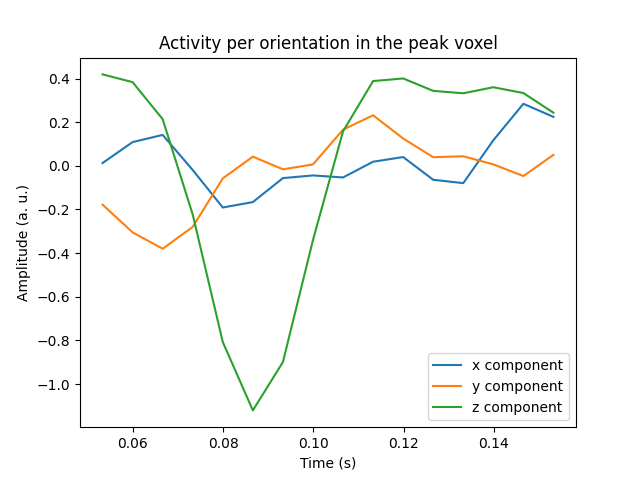## Morph the output to fsaverage#

We can also use volumetric morphing to get the data to fsaverage space. This is for example necessary when comparing activity across subjects. Here, we will use the scalar beamformer example. We pass a `mne.SourceMorph` as the `src` argument to `mne.VolSourceEstimate.plot`. To save some computational load when applying the morph, we will crop the `stc`:

```fetch_fsaverage(subjects_dir)  # ensure fsaverage src exists
fname_fs_src = subjects_dir / "fsaverage" / "bem" / "fsaverage-vol-5-src.fif"

morph = mne.compute_source_morph(
src,
subject_from="sample",
src_to=src_fs,
subjects_dir=subjects_dir,
niter_sdr=[5, 5, 2],
niter_affine=[5, 5, 2],
zooms=7,  # just for speed
verbose=True,
)
stc_fs = morph.apply(stc)
del stc

stc_fs.plot(
src=src_fs,
mode="stat_map",
initial_time=0.085,
subjects_dir=subjects_dir,
clim=dict(kind="value", pos_lims=lims),
verbose=True,
)
```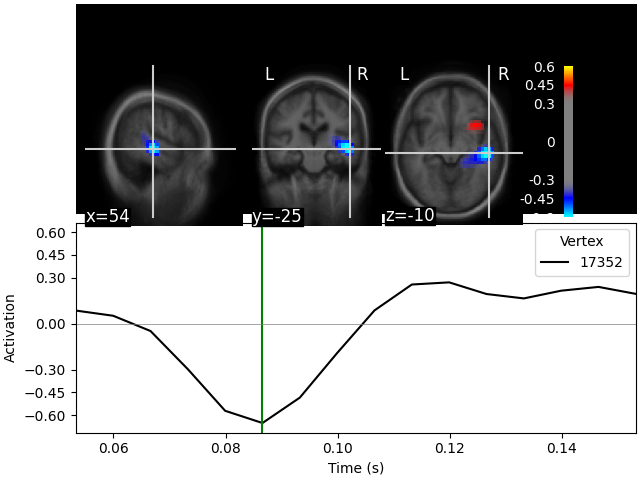```0 files missing from root.txt in /home/circleci/mne_data/MNE-sample-data/subjects
0 files missing from bem.txt in /home/circleci/mne_data/MNE-sample-data/subjects/fsaverage
[done]
Volume source space(s) present...
Computing registration...
Reslicing to zooms=(7.0, 7.0, 7.0) for translation ...
Optimizing translation:
Optimizing level 2 [max iter: 5]
Optimizing level 1 [max iter: 5]
Optimizing level 0 [max iter: 2]
Translation:   22.7 mm
R²:            92.1%
Optimizing rigid:
Optimizing level 2 [max iter: 5]
Optimizing level 1 [max iter: 5]
Optimizing level 0 [max iter: 2]
Translation:   22.7 mm
Rotation:      19.7°
R²:            96.3%
Optimizing affine:
Optimizing level 2 [max iter: 5]
Optimizing level 1 [max iter: 5]
Optimizing level 0 [max iter: 2]
R²:            96.6%
Optimizing sdr:
R²:            98.3%
[done]

0%|          | Time : 0/16 [00:00<?,       ?it/s]
6%|▋         | Time : 1/16 [00:00<00:01,    7.71it/s]
12%|█▎        | Time : 2/16 [00:00<00:01,    8.31it/s]
19%|█▉        | Time : 3/16 [00:00<00:01,    8.91it/s]
25%|██▌       | Time : 4/16 [00:00<00:01,    9.25it/s]
31%|███▏      | Time : 5/16 [00:00<00:01,    9.47it/s]
38%|███▊      | Time : 6/16 [00:00<00:01,    9.62it/s]
44%|████▍     | Time : 7/16 [00:00<00:00,    9.73it/s]
50%|█████     | Time : 8/16 [00:00<00:00,    9.81it/s]
56%|█████▋    | Time : 9/16 [00:00<00:00,    9.88it/s]
62%|██████▎   | Time : 10/16 [00:01<00:00,    9.93it/s]
69%|██████▉   | Time : 11/16 [00:01<00:00,    9.97it/s]
75%|███████▌  | Time : 12/16 [00:01<00:00,   10.00it/s]
81%|████████▏ | Time : 13/16 [00:01<00:00,   10.04it/s]
88%|████████▊ | Time : 14/16 [00:01<00:00,   10.06it/s]
94%|█████████▍| Time : 15/16 [00:01<00:00,   10.08it/s]
100%|██████████| Time : 16/16 [00:01<00:00,   10.10it/s]
100%|██████████| Time : 16/16 [00:01<00:00,   10.00it/s]
Fixing initial time: 0.085 s
Showing: t = 0.087 s, (55.0, -25.0, -10.0) mm, [27, 18, 13] vox, 17352 vertex
```